# How to draw ODEs in Simulink

I remember while learning Simulink, drawing ordinary differential equations was one of the early challenges. Eventually I discovered a few steps that make it easier. First, rewrite the equations as a system of first order derivatives. Second, add integrators to your model, and label their inputs and outputs. Third, connect the terms of the equations to form the system.

Example: Mass-Spring-Damper

The mass-spring-damper system provides a nice example to illustrate these three steps. Let’s look at the equation for this system: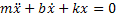The position of the mass is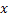, the velocity is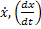, and the acceleration is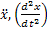.

Express the system as first order derivatives

To rewrite this as a system of first order derivatives, I want to substitute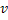for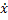, and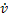for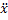. Then I can identify my two states as position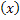and velocity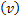. The equation becomes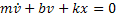And this is rewritten at two first derivatives: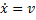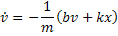Velocity and position are the states of my system. When thinking about ODEs, states equal integrator blocks.

Add one integrator per state, label the input and output

I always make a point to write the equations as an annotation on my diagram. I refer to this as I add blocks to the canvas. Here are the two integrators for the mass-spring-damper system.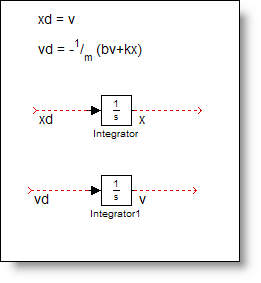I draw signals from the ports and label inputs as the derivative (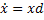), and the output is the state variable.

Connect the terms to form the system

The first connection is easy,, so I connect the output of the velocity integrator to the input of the position integrator. When this happens, aligning the integrators in the diagram shows that you have a second order system.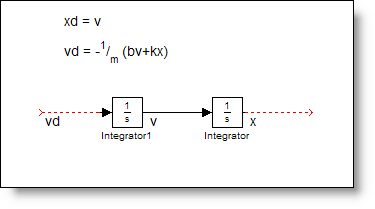To implement the second equation, I add gains and sums to the diagram and link up the terms.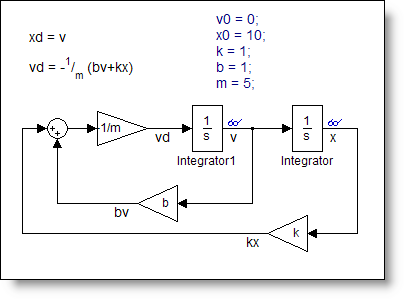The final step, initial conditions

Modeling differential equations require initial conditions for the states in order to simulate. The initial states are set in the integrator blocks. Think of these as the initial value for v and x at time 0. The ODE solvers compute the derivatives at time zero using these initial conditions and then propagate the system forward in time. I used an annotation to record these initial conditions, v0 = 0, and x0 = 10.

Simulating the model for 50 seconds produces the following trace for x (blue) and v (red).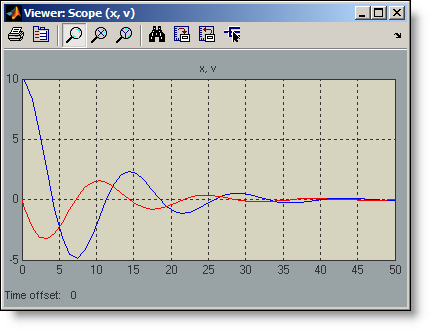Over time, I have become more comfortable in converting from equations to models, and I do not always rewrite the states. I think that fundamentally I still follow the same process:

1. Re-express the system in terms of state derivatives
2. Add integrators and label the inputs and outputs
3. Connect up the equations
4. Set initial conditions